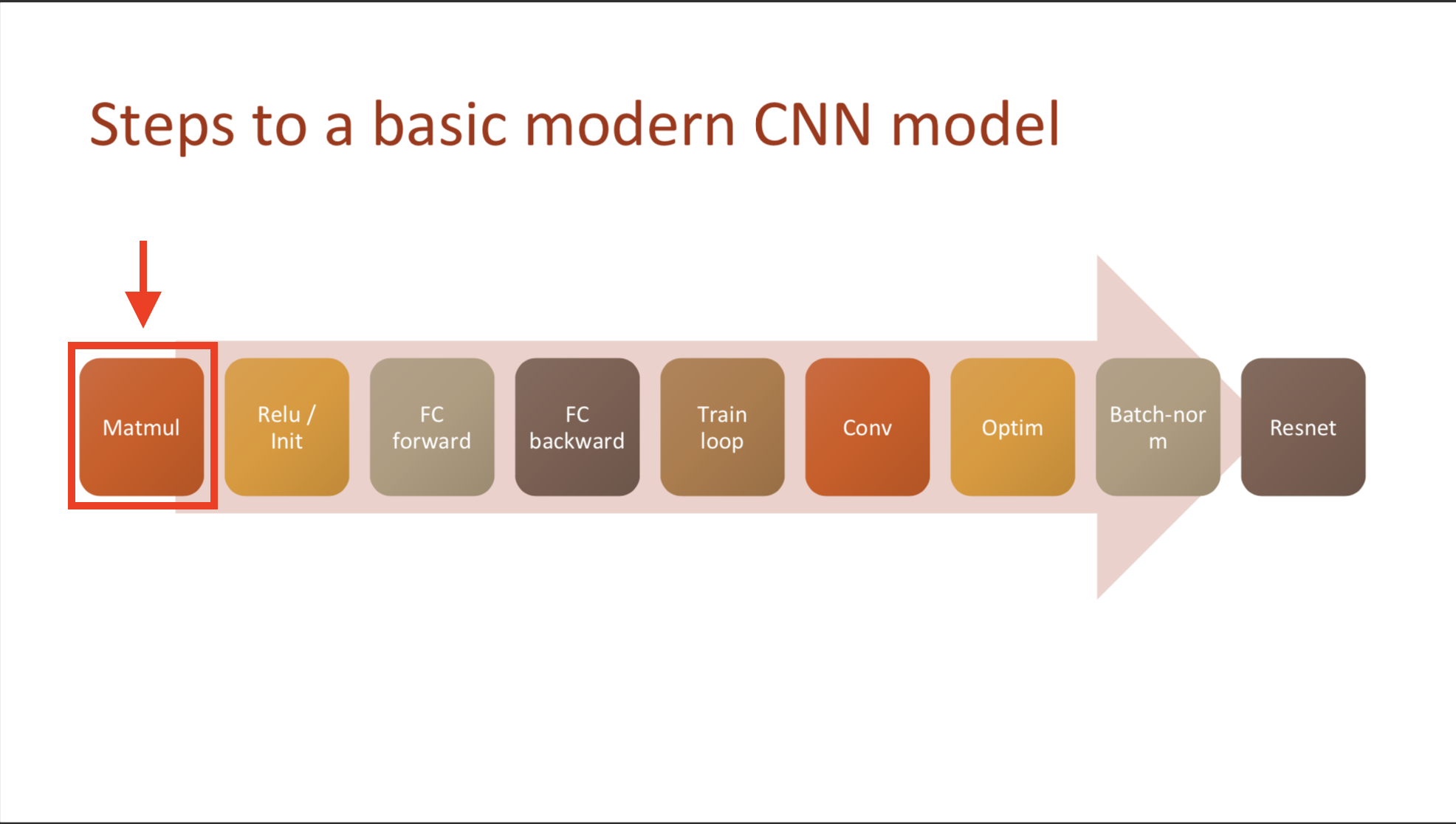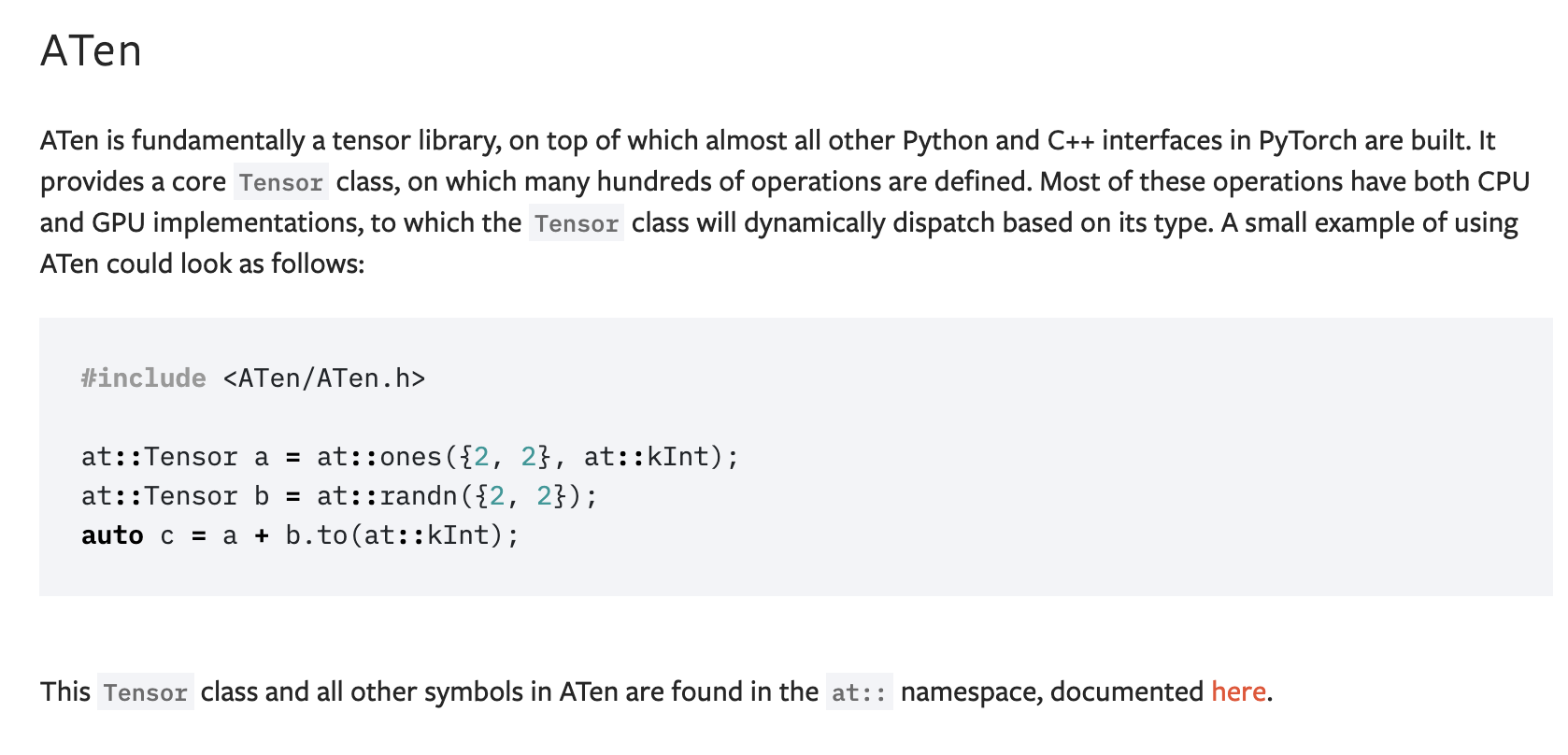# What is the meaning of 'deep-learning from foundations?'

Feb 29, 2020 · 4 mins readShare this

This note is divided into 4 section.

” Lecture 08 - Deep Learning From Foundations-part2 “

I don’t know if you read this article, but I heartily appreciate Rachael Thomas and Jeremy Howard for providing these priceless lectures for free

### Homework

• Review concepts 16 concepts from Course 1 (lessons 1 - 7) (1) Affine Functions & non-linearities; 2) Parameters & activations; 3) Random initialization & transfer learning; 4) SGD, Momentum, Adam; 5) Convolutions; Batch-norm; 6) Dropout; 7) Data augmentation; 8) Weight decay; 9) Res/dense blocks; 10) Image classification and regression; 11)Embeddings; 12) Continuous & Categorical variables; 13) Collaborative filtering; 14) Language models; 15) NLP classification; 16) Segmentation; U-net; GANS)

## What is going on in this course?

### What is ‘from foundations’?

1) Recreate fast.ai and Pytorch

2) using pure python

• Evade Overfitting

Overfit : validation error getting worse training loss < validation loss

• Know the name of the symbol you use

find in this page if you don’t know the symbol that you are using or just draw it here (run by ML!)

### Steps to a basic modern CNN model

1) Matrix multiplication -> 2) Relu/Initialization -> 3) Fully-connected Forward -> 4) Fully-connected Backward -> 5) Train loop -> 6) Convolution-> 7) Optimization -> 8) Batchnormalization -> 9) Resnet

## Library development using jupyter notebook

what is assers?

### jupyter notebook certainly can make module

• There will be #export tag that Howard (and we) want to extract
• special notebook2script.py will detect sign of #expert and convert following into python module
• and test it

test\_eq(TEST,'test')test\_eq(TEST,'test1')

• what is run_notebook.py?
• when you want to test your module in command line interface

 !python run\_notebook.py 01_matmul.ipynb

• Is there any difference between 1) and 2)?

1) test -> test01 2) test01 -> test

#TODO I don’t know yet

• look into run_notebook.py, package fire Jeremy used. What is that?

read and run the code in a notebook, and in the process, Jeremy made Python Fire library called!shockingly, fire takes any kind of function and converts into CLI command.

fire library was released by Google open source, Thursday, March 2, 2017

• Get data

• pytorch and numpy are pretty much same.
• variable c explains how many pixels there are in in MNIST, 28 pixels
• PyTorch’s view() method: torch function that manipulating tensor, and squeeze() in torch & mathmatical operation similar function
• Rao & McMahan said usually this functions result in feature vector.
• In part 1, you can use view function several times.

• Initial python model

• Which is Linear, like $Xw$(weight)$+a$(bias) $= Y$

• If you don’t know hou to multiple matrix, refer this site matmul visulization site

• How many time spends if we we use pure python
• function matmul, typical matrix multiplication function, takes about 1 second for calculating 1 single train data! (maybe assumed stochastic, 5 data points in validation)

• it takes about 11.36 hours to update parameters even single layer and 1 iteration! (if that was my computer, it would be 14 hours..)🤪

• THIS is why we need to consider ‘time’&’space’

This is kinda slow - what if we could speed it up by 50,000 times? Let’s try!

## Elementwise ops

### How can we make python faster?

• If we want to calculate faster, then do remove pythonic calcuation, by passing its computation down to something that is written something other than python, like pytorch.
• According to PyTorch doc it uses C++ (via ATen), so we are going to implement that function with python.#### What is element wise operation?

• items makes a pair, operate corresponding component